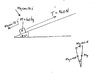# Does AP count as College?

Did I do this problem right? I didn't use some of the info...

Oh well... if it doesn't, it's moveable. :)

I'm new on here, and I was wondering if someone could help me with my physics HW; And forgive me if I don't get it right away. :)

Here's the problem...
A 40kg. wagon is towed up a hill inclined at 18.5(degrees) with respect to the horizontal. The tow rope is parallel to the incline and has a tension of 140N in it. Assume that the wagon starts from rest at the botton of the hill and neglect friction. How fast is the wagon going after moving 80m. up the hill?

I tried doing this one, and well...

m=40kg.
delta(x)=80m.
Angle=18.5
Force(net)=140N
vf=?

Just stating the known, and then I...

v(fin)^2=v(ini)^2 + 2a(delta)x
vf^2 = 0 +160a

F(net)=ma
140=40a
a=3.5

in which case...

vf^2= 160(3.5)
vf^2= 560
vf = 23.66

So, that's what I did; I'm sure it's wrong, just because I didn't use the angle.
So... that's my question... what did I do wrong? Or did I do it right?

Thanks a bundle!
P.S. Heheh, I have a whole lot of physics "?"s that I don't understand...

Last edited by a moderator:

Homework Helper
TheRhinoMan said:
...

F(net)=ma
140=40a

...
The wagon is on an incline. So the accelerating force isn't 140N.
You have to take away the component of the wagon's weight acting down the slope.

OOOooohhhh...
I see it now, Thanks Fermat!

I called a friend last night, and he was able to help me with this problem... but I didn't understand his reasoning. Now I do; thanks!.

So... Basically, I had to make a right triangle, force of gravity being the hypotenuse (sp?), normal force being the second largest side, and the base (or smallest side) being, essentially, the force reacting against the wagon as it goes up.

The wagon needed to overcome that force and then some in order to accelerate up the hill...
Yay! I think I get it. :) I'll try another one similar to it, and if I still don't get it, I'll be back. :D

Thanks for the help, fermat!

TheRhinoMan

Homework Helper
Your setup should look something like in the attachment.

The force acting against the wagon is its component down the slope, i.e. Mg.sin(18.5).
So, the accelerating force is,

F = T - Mg.sin(18.5)

#### Attachments

•rhinoman.jpg
18.3 KB · Views: 426